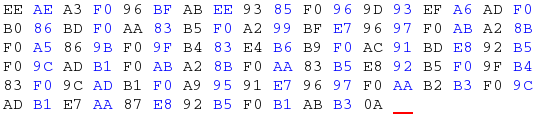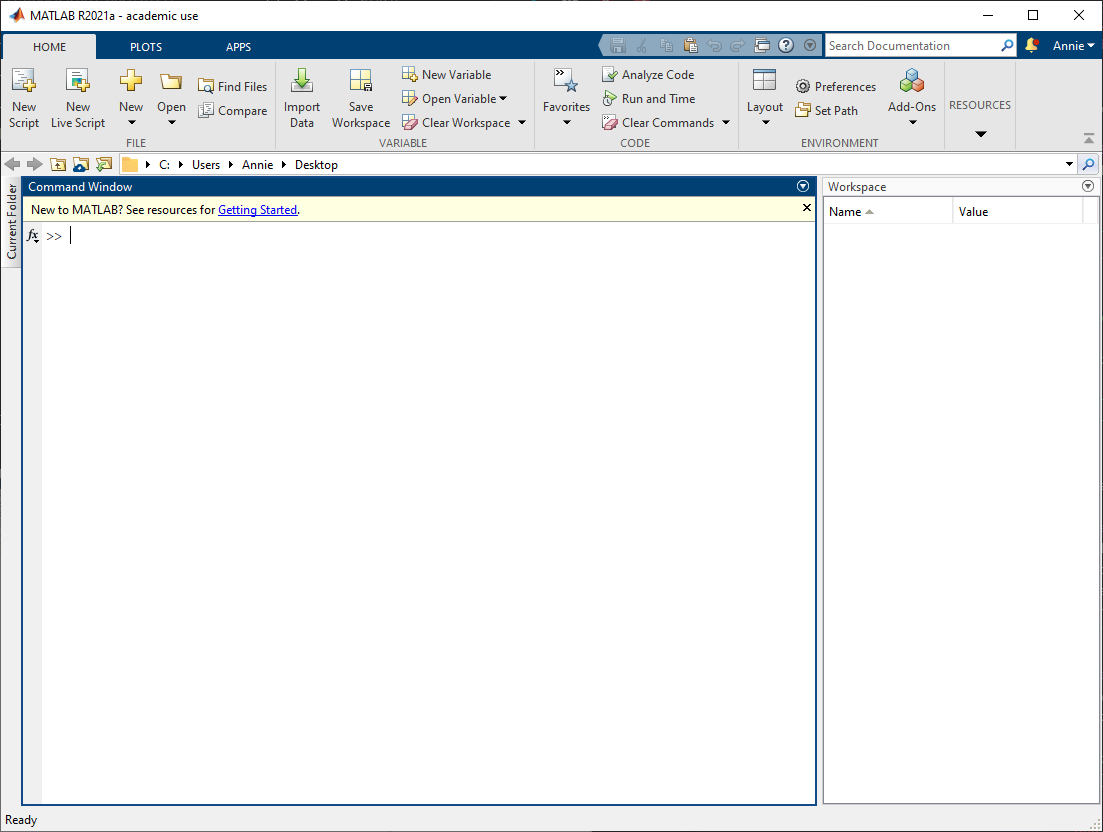# Path to a crypto master, the engineer way

Published onWho knew I’d be writing a crypto writeup.

## The Beginning

The challenge provides a SageMath bit of code, as well as a cipher text.

``````def encrypt(msg, f):
return ''.join(chr(f.substitute(c)) for c in msg)

P.<x> = PolynomialRing(ZZ)
f = 13*x^2 + 3*x + 7

enc = encrypt(FLAG, f)
print(enc)
``````That’s some messy looking cipher text…

### Understanding the sage

Thankfully the SageMath here is nice and short. All that we need to know, is that each character of the flag is thrown into the encryption function `f`. So to reverse it, we just do the opposite.
Since the values were obtained by substituting each character into `13*x^2 + 3*x + 7`, to get x back, we solve `13*x^2 + 3*x + 7 = <encrypted num>`.

### Neat-ify the cipher text

Those bytes looked a little unpleasant to me, so I decided to turn them into their appropriate unicode values.

``````txt = open("output.txt").read()
nums = []

for chr in txt:
nums.append(ord(chr))

print(nums)
``````

`nums = [60323, 94187, 58565, 91987, 63917, 197053, 172277, 140927, 30103, 178315, 151963, 130307, 19897, 181373, 33973, 117617, 178315, 172277, 33973, 130307, 117617, 169297, 30103, 175283, 117617, 31367, 33973, 203507, 10]`
Now that’s much better. Now to simply reverse the values and …

## Oh no

### An unnatural call

I then felt a disturbance in the air. My computer was absorbed by a bright, blinding light. When my senses recovered I saw this:I guess I have no choice now. The call of engineers can not be ignored.

### An unholy solve

Matlab thankfully has a very simple way of solving quadratic equations. Using the `root()` function, in addition to rounding, I summoned the following code:

``````nums = [60323, 94187, 58565, 91987, 63917, 197053, 172277, 140927, 30103, 178315, 151963, 130307, 19897, 181373, 33973, 117617, 178315, 172277, 33973, 130307, 117617, 169297, 30103, 175283, 117617, 31367, 33973, 203507, 10];
results = "";
for i = 1:length(nums)
results(i) = char(round(max(roots([13 3 (7-nums(i))]))));
end

sprintf("%s",results)
``````

And just like that, the blinding light disappeared, and a flag emerged… `DUCTF{sh0uld'v3_us3d_r0t_13}`

What have I done…